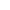Go to content
Where two or three events occur, following on from each other, the various outcomes can be illustrated clearly on a tree diagram . This is particularly useful when later events depend in some way on the outcomes of previous events.## Summary/Background

A tree diagram is used to illustrate probabilities when two things happen by displaying outcomes and their probabilities on the branches. For example when two coins are spun, or if someone takes a counter from one bag followed by a counter from another bag, then the possibilities are shown on a tree diagram. The diagram shows all the possible outcomes. Usually the probability of each individual outcome is marked on each branch.You can get a better display of the maths by downloading special TeX fonts from jsMath. In the meantime, we will do the best we can with the fonts you have, but it may not be pretty and some equations may not be rendered correctly.

## Glossary

### tree

A connected graph with no cycles.

### union

The union of two sets A and B is the set containing all the elements of A and B.

Full Glossary List

## This question appears in the following syllabi:

SyllabusModuleSectionTopicExam Year
AQA GCSE (9-1) Foundation (UK)P: ProbabilityP8: Combined EventsTree Diagrams-
CIE IGCSE (9-1) Maths (0626 UK)8 ProbabilityB8.5 Simple Combined EventsTree Diagrams-
Edexcel GCSE (9-1) Foundation (UK)P: ProbabilityP8: Combined EventsTree Diagrams-
GCSE Higher (UK)ProbabilityProbabilityTree diagrams-
OCR GCSE (9-1) Foundation (UK)11: Probability11.02d: Tree DiagramsTree Diagrams-
Universal (all site questions)PProbabilityTree diagrams-